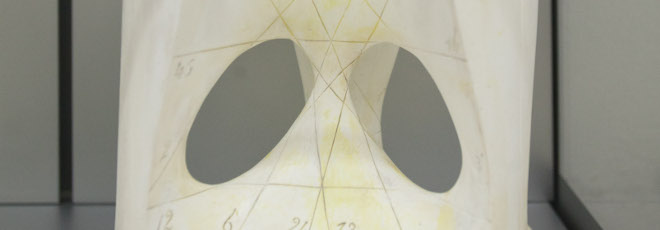## “On the structure of weak solutions to Burgers equation”

Venerdì 22 Maggio 2020, ore 15:00 - Zoom - Elio Marconi (University of Basel)

Abstract

We consider the Burgers equation: $$u_t+ (u^2/2)_x =0 \qquad (1)$$

The well-posedness of the corresponding Cauchy problem in the class of bounded entropy solutions and their regularity are by now classical.

Nevertheless there are situations where a more general class of weak solutions to $(1)$ arises, namely weak solutions with finite entropy production.

In contrast with bounded entropy solutions, weak solutions with finite entropy production may fail to have locally bounded variation, but they share with BV functions most of the fine properties.

The goal of this talk is to introduce the notion of “Lagrangian representation”, which extends the classical method of characteristics, and to adoperate it to investigate the structure of weak solutions with finite entropy production to $(1)$.